# TensorBoard Tutorial in Keras for Beginner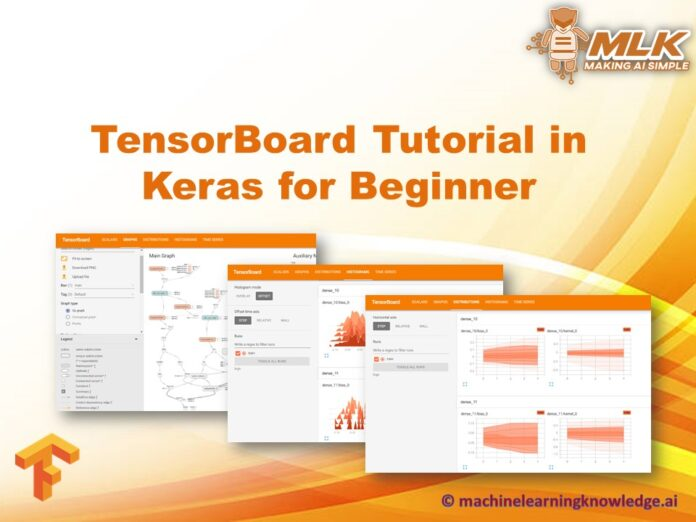## Introduction

In this article, we will go through the tutorial for TensorBoard which is a visualization tool to understand various metrics of your neural network model and the training process. We will first explain what is TensorBoard and how to install it. Then, we will show you an example of how to use Tensorboard using Keras and go through various visualizations.

## What is TensorBoard?

TensorBoard is a visualization web app to get a better understanding of various parameters of the neural network model and its training metrics. Such visualizations are quite useful when you are doing experiments with neural network models and want to keep a close watch on the related metrics. It is open-source and is a part of the Tensorflow group.

Some of the useful things you can do with TensorBoard includes –

• Visualize metrics like accuracy and loss.
• Visualize model graph.
• Visualize histograms for weights and biases to understand how they change during training.
• Visualize data like text, image, and audio.
• Visualize embeddings in lower dimension space.

## TensorBoard Tutorial (Keras)

Here we are going to use a small project to create a neural network in Keras for Tensorboard Tutorial. For this, we are going to use the famous MNIST handwritten digit recognition dataset.

Since this is a TensorBoard tutorial, we will not explain much about the data preprocessing and neural network building process. To understand more details about working with MNIST handwritten digit dataset you can check below tutorial –

### i) Install TensorBoard

You can install TensorBoard by using pip as shown below –

`pip install tensorboard`

### ii) Starting TensorBoard

The first thing we need to do is start the TensorBoard service. To do this you need to run below in the command prompt. –logdir parameter signifies the directory where data will be saved to visualize TensorBoard. Here we have given the directory name as ‘logs’.

` tensorboard --logdir logs`

This will start the TensorBoard service at the default port 6066 as shown below. The TesnorBoard dashboard can be accessed as http://localhost:6006/

Output:

```Serving TensorBoard on localhost; to expose to the network, use a proxy or pass --bind_all
TensorBoard 2.5.0 at http://localhost:6006/ (Press CTRL+C to quit)```

In Jupyer notebook, you can issue the following command in the cell

`%tensorboard --logdir logs`

We will quickly import the required libraries for our example. (Do note these libraries have nothing to do with TensorBoard but are needed for building the neural network of our example.)

### v) Preprocessing

We will now preprocess the data by normalizing it between 0 to 1 and then flattening it.

In :
```X_train = X_train / 255
X_test = X_test / 255

X_train_flattened = X_train.reshape(len(X_train), 28*28)
X_test_flattened = X_test.reshape(len(X_test), 28*28)
```

### xi) Create and Compile the Model

Now we create and compile a simple neural network model consisting of just one input layer, one hidden layer of 100 neurons, and one output layer. All other configurations are standard.

```model = keras.Sequential([
keras.layers.Flatten(input_shape=(28, 28)),
keras.layers.Dense(100, activation='relu'),
keras.layers.Dense(10, activation='sigmoid')
])

loss='sparse_categorical_crossentropy',
metrics=['accuracy'])

```

### vii) Creating Callback Object

This is where we need to draw our attention while working with TensorBoard. We have to create a Keras callback object for TensorBoard which will help to write logs for TensorBoard during the training process.

Please do note that the parent path for log_dir below should be the same as the logdir value we gave while starting the TensorBoard service in the second step.

```tb_callback = tf.keras.callbacks.TensorBoard(log_dir="logs/", histogram_freq=1)
```

### viii) Training Model

Finally, we start the training of the model by using fit() function. We train it for 5 epochs and do notice that we have also passed the callback object that we created in the previous step.

`model.fit(X_train, y_train, epochs=5,callbacks=[tb_callback])`
```Epoch 1/5
1875/1875 [==============================] - 3s 2ms/step - loss: 0.2773 - accuracy: 0.9206
Epoch 2/5
1875/1875 [==============================] - 3s 2ms/step - loss: 0.1255 - accuracy: 0.9627
Epoch 3/5
1875/1875 [==============================] - 3s 2ms/step - loss: 0.0881 - accuracy: 0.9738
Epoch 4/5
1875/1875 [==============================] - 3s 2ms/step - loss: 0.0668 - accuracy: 0.9793
Epoch 5/5
1875/1875 [==============================] - 3s 2ms/step - loss: 0.0528 - accuracy: 0.9840```

### ix) Visualization Model in Tensorboard

We can now go to the TensorBoard dashboard that we started in the first step and see what all visualizations it has to offer. The visualizations mostly depend on what data you have logged for TensorBoard. Depending on the logged data corresponding TensorBoard plugins get activated and you can see them by selecting ‘Inactive’ dropdown in the top right corner of the dashboard.

Let us see the visualizations available in our example.

#### Scalars

It shows visualizations for accuracy and loss in each epoch during the training process. And when you hover the graph it shows more information like value, step, time.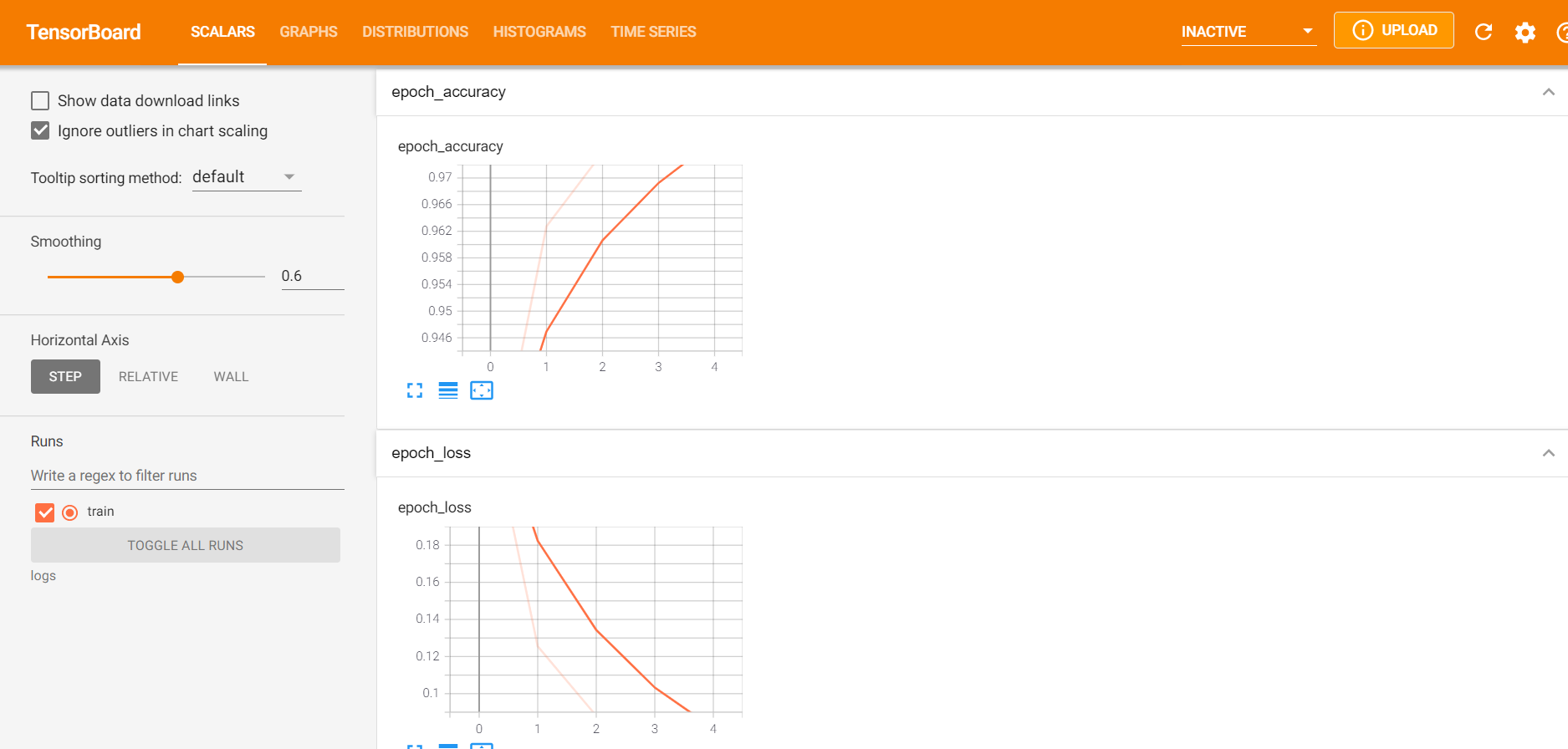#### Graph

The neural network model is essentially computational graphs in TensorFlow Keras and it can be visualized in this section.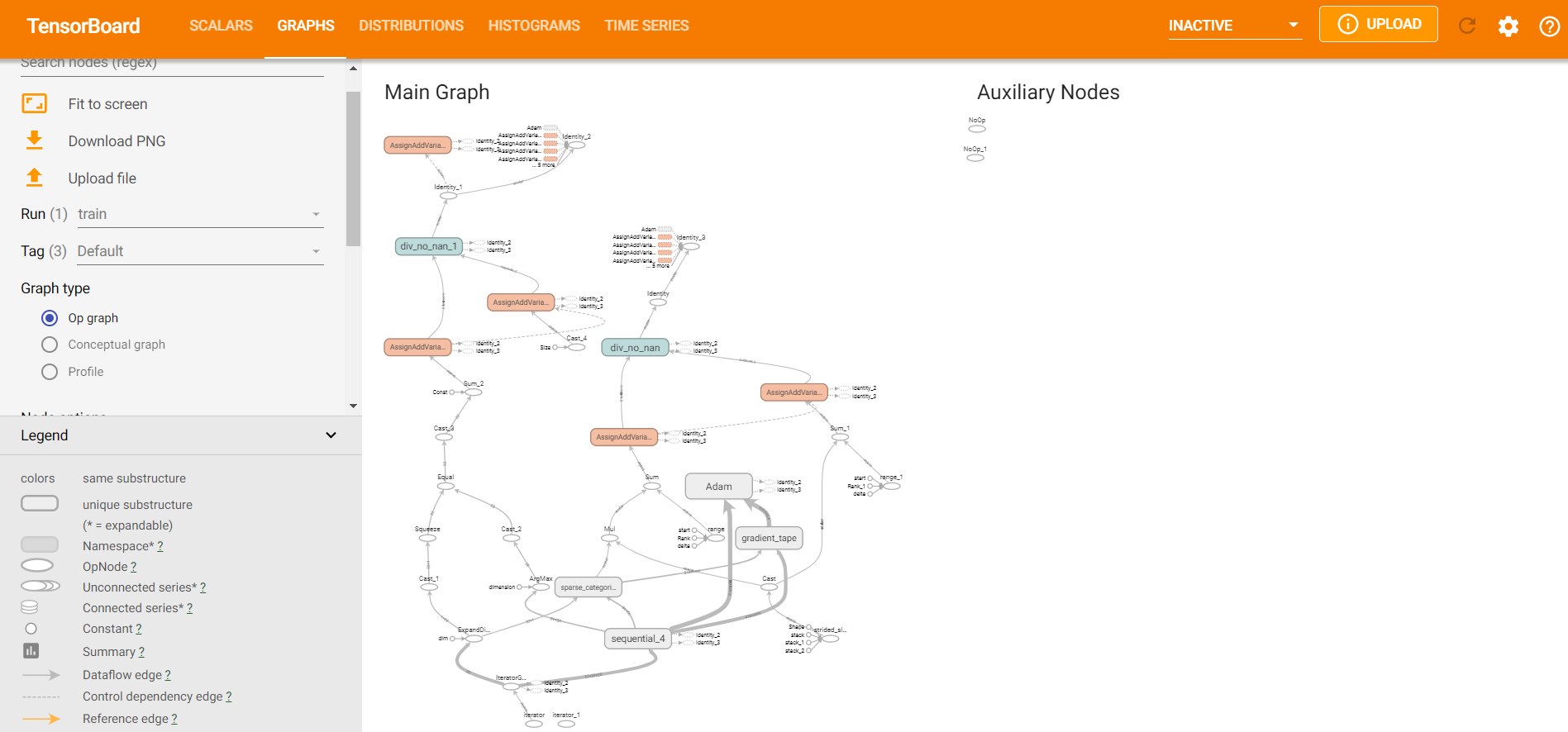#### Distribution

This section shows the change of weights and biases over the time period of training.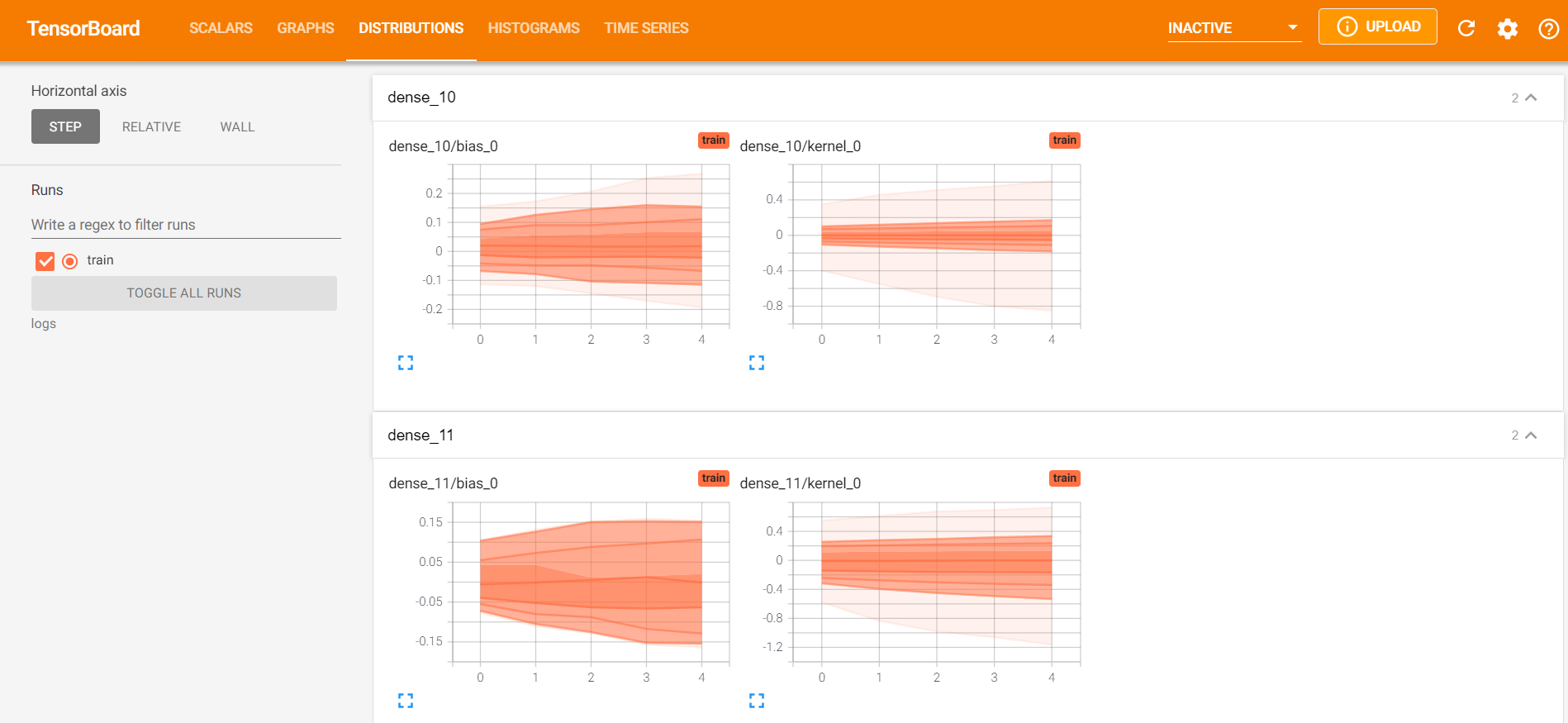#### Histograms

This also shows the distribution of weights and bias over time in a 3D format.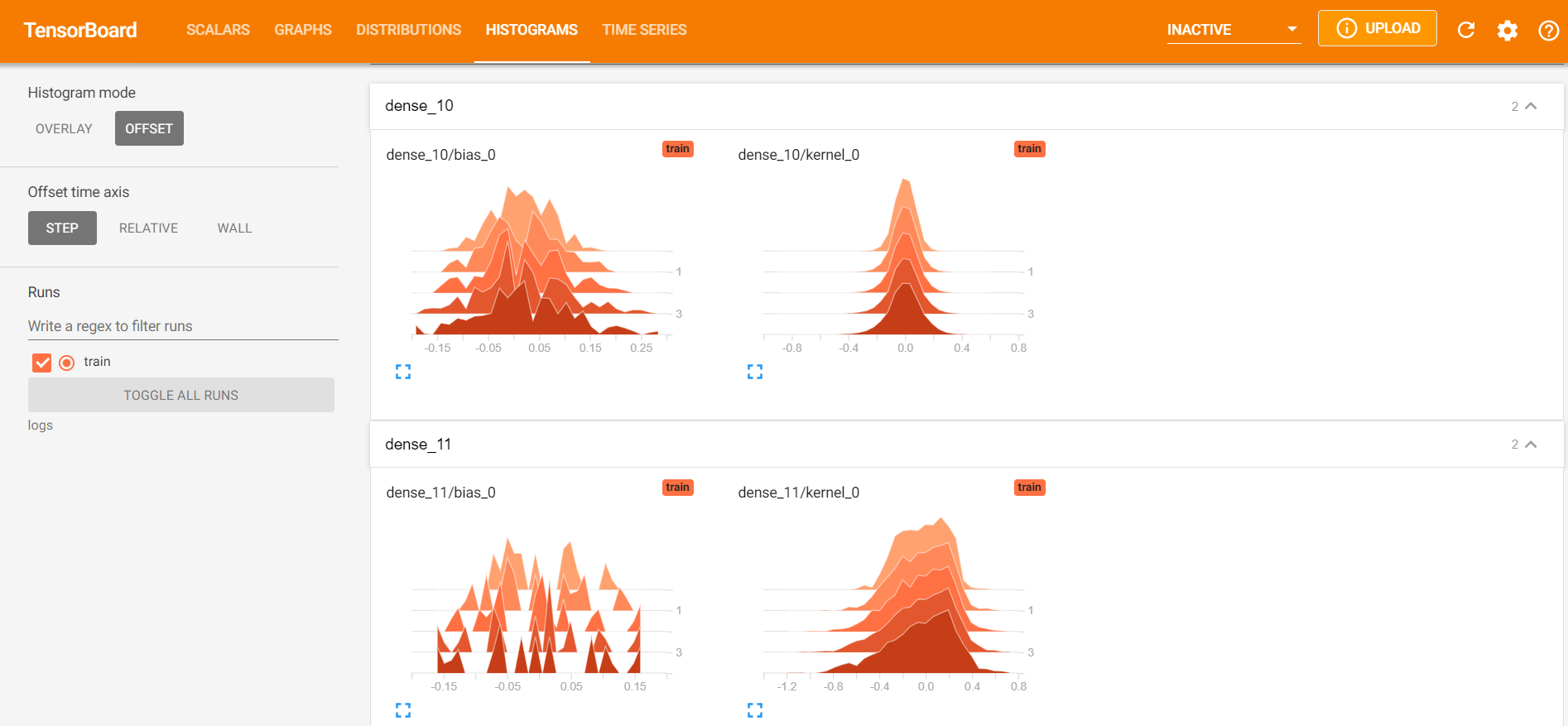### x) Comparing Different Models in TensorBoard

Creating a good Neural Network is not a straightforward job and requires multiple runs to experiment with various parameters. With TensorBoard, you can visualize the performance of all the model runs in the dashboard and compare them easily.

For this, we will create the logs of training in different subfolders inside the main folder. The below example will help you understand better.

In the first run, we create the Keras callback object of TensorBoard whose logs are going to be saved in the ‘run1’ folder inside the main logs folder.

```tb_callback = tf.keras.callbacks.TensorBoard(log_dir="logs/run1", histogram_freq=1)
model.fit(X_train, y_train, epochs=5, callbacks=[tb_callback])```

In the second run, we give the log path as run2 as shown below.

```tb_callback = tf.keras.callbacks.TensorBoard(log_dir="logs/run2", histogram_freq=1)
model.fit(X_train, y_train, epochs=5, callbacks=[tb_callback])```

Now when we see the TensorBoard dashboard, it will show information for both the runs in orange and blue lines for accuracy and loss graph.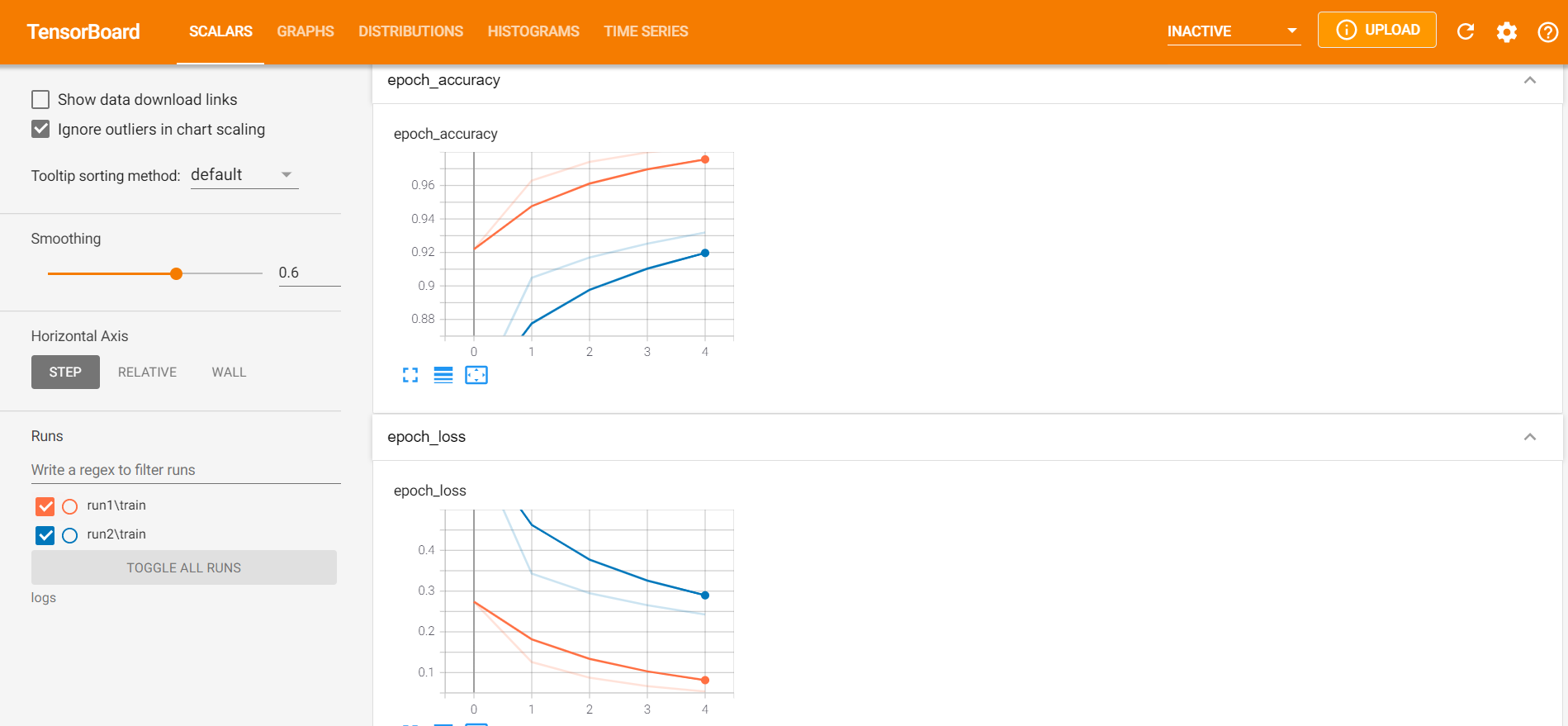## Conclusion

Hope you found this article quite useful where we gave a small introductory tutorial on TensorBoard for beginners. We understood how to install and start the TensorBoard dashboard, along with various visualizations with the help of an example in Keras.